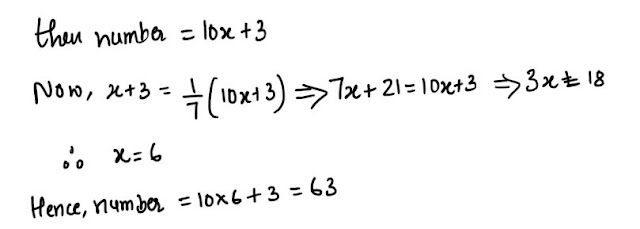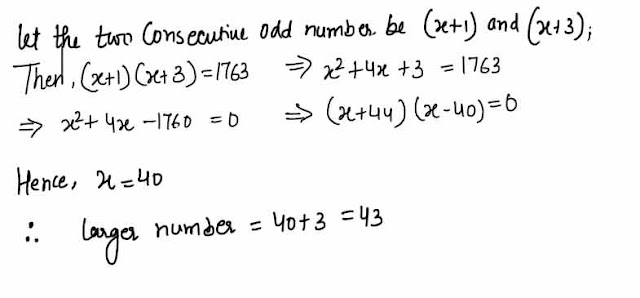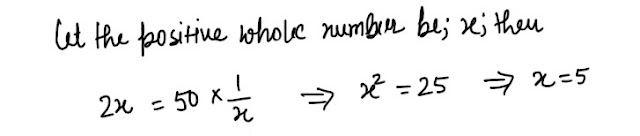# Number System : 10 Very Important Questions for Upcoming Exams#### Ques 1.

Three times the first of three consecutive odd integers is 3 more than twice the third. What is the third integer ?
(a) 15
(b) 13
(c) 11
(d) 9
Ans 1. Let the three consecutive odd number be ( x + 1) ,( x+ 3) , ( x+ 5) ;
then, 3 ( x+ 1) = 2 ( x + 5) + 3 =>   x = 10
Hence, the second number  = 10 + 5 = 15

#### Ques 2.

A Number of two  digits has 3 for its unit's digit,and the sum digit is 1/7 of the number itself.What is the number ?
(a) 73
(b) 63
(c) 53
(d) 43
Ans 2. Let the digit at the ten's place be x;#### Ques 3.

The product of three consecutive even numbers when divided by 8 is 720.What is the product of their square roots ?
(a) 120
(b) 42/10
(c) 24/10
(d) 12/10
Ans 3.#### Ques 4.

In a two number, the product of the digits is 8. when 18 is added to the number,then the digits are reversed.What is the number ?
(a) 81
(b) 42
(c') 24
(d) 18
Ans 4. Let the number be 10x + y; then#### Ques 5.

If i added to the denominator of a  fraction, the fraction becomes 1/2. If is added to the numerator of the fraction,the fraction becomes I. What is the fraction ?
(a) 3/2
(b) 3/4
(c) 2/3
(d) 1/3
Ans 5.#### Ques 6.

What is the greater of the two numbers whose product is 640, if the sum of the two numbers exceeds their difference by 32?
(a) 45
(b) 50
(c) 55
(d) 40
Ans 6.  Let the  two number be x and y; then#### Ques 7.

The product of two consecutive odd numbers is 1763.What is the larger number /
(a) 43
(b) 39
(c) 41
(d) 37
Ans 7.#### Ques 8.

Two numbers are such that their sum is  16 and their product  is 55. What is the sum of their reciprocals ?
(a) 27/55
(b) 16/55
(c) 37/45
(d) 38/85
Ans 8.#### Ques 9.

The  double of a positive whole number is 50 times the value of its reciprocal.What is the number ?
(a) 20
(b) 10
(c) 5
(d) 2
Ans 9.#### Ques 10.

Two numbers are such that their product, their sum and their difference are in the ratio of 6 : 2 : 1 respectively .Their sum is :
(a) 16
(b) 20
(c) 24
(d) 30
Ans 10.==>> Follow Quantitative Aptitude Shortcuts here